WEBINAR
Numerical Analysis of Magnetic Pulse Welding Process
Thursday, October 5, 2023
Time
SESSION 1
SESSION 2
CEST (GMT +2)
03:00 PM
08:00 PM
EDT (GMT -4)
09:00 AM
02:00 PM
HOME / Applications / Inductance Capacitance and Resistance of a PCB structure

# Inductance Capacitance and Resistance of a PCB structure

Used Tools:## Parasitic parameters of a PCB

EMI, or electromagnetic interference, is an undesirable electromagnetic noise from a device or a system that interferes with the normal operation of neighboring devices or systems.
The basic process of EMI modeling and prediction requires the extraction of parasitic parameters of a PCB and circuit components to build high frequency circuit models.

## Parasitic capacitance, parasitic resistance, and parasitic inductance

In electronic design automation (EDA), parasitic extraction is the calculation of the parasitic effects in both the designed devices and the required wiring interconnects of an electronic circuit: parasitic capacitance, parasitic resistance, and parasitic inductance. In this article, we illustrate the use of EMS to calculate these circuit parameters and compare the results to published data .

## Soildworks PCB model

A PCB structure shown in Figure 1, contains two copper traces on a 4-oz FR4 PCB square board and a 5 mils thickness copper Ground. Some parameters used in simulation are given below where all dimensions are in mils.
Conductivity of Copper = 5.8 107S/m
Relative Permittivity of FR4 = 4.4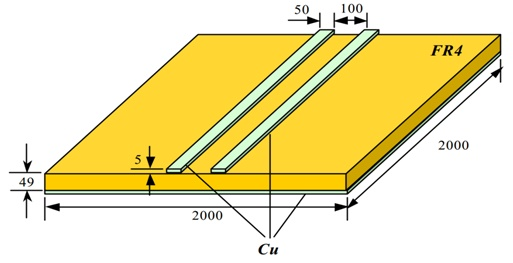Figure 1 -  A PCB structure used for simulation where all dimensions are in mils

## Capacitance calculation

To compute the parasitic capacitance of the PCB structure, shown in Figure 1, the Electrostatic module is invoked. Figure 2 shows the model and the mesh for the PCB structure.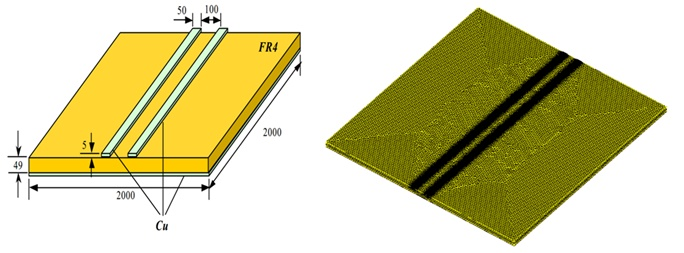Figure 2 - Model and mesh of the PCB structure

## Three floating conductors

To account for the capacitance of a given conductor, it is assigned a floating boundary condition, including the ground plane, in EMS.  As a result, this PCB structure has three floating conductors, i.e. the left and right traces as well as the ground plane.
The EMS capacitance results and that of the Reference  are shown in Figure 3 and Table 1.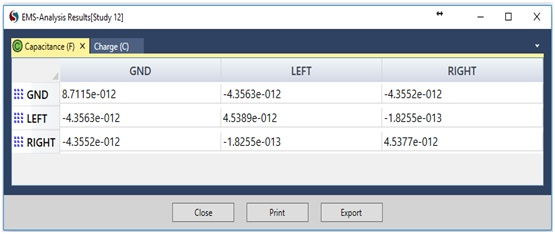Figure 3 - Parasitic capacitance computed by EMS

 Reference  EMS The capacitance between the left trace and the ground plane -4.3047 pF -4.3563 pF The capacitance between the right trace and the ground plane -4.3046 pF -4.3552 pF The capacitance between the two copper traces -0.1673 pF -0.1825 pF
Table 1 - Capacitance results of EMS compared to Reference 

## DC Inductance & DC Resistance calculation

To compute the DC resistance and inductance of the PCB structure, shown in Figure 1, the EMS Magnetostatic module is invoked.  To compute the DC inductance and DC resistance of the copper traces, they are modeled as coils. The DC inductance and DC resistance results obtained by EMS and compared to Reference , are shown below: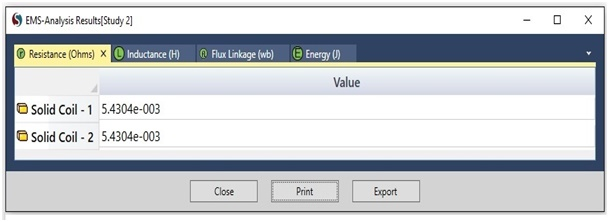Figure 4 - DC Resistance computed by EMS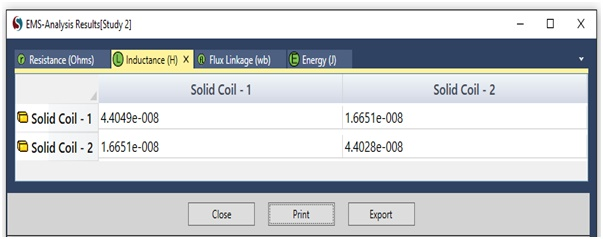Figure 5 - DC Inductance computed by EMS

DC loop Inductance is obtained by the following formula:
L Loop = L11+L22- 2*M12 ; where L11, L22: self inductances; M12: mutual inductance

 Reference  EMS DC resistance 5.4304 m Ohm 5.4304 m Ohm DC Loop inductance 50.742 n Henry 54.775 n Henry
Table 2 - DC resistance and DC inductance results produced by EMS and compared to Reference 

## AC Inductance & AC Resistance calculation

In addition to DC inductance and resistance calculation, EMS is equipped with AC Magnetic and eddy current capabilities which are used to compute the AC resistance and AC loop inductance for the PCB structure at hand at the frequencies: 1Khz, 2Khz, 5Khz, 10 KHz, 20 KHz, 50 KHz, 100 KHz, 200 KHz, 500 KHz, 1 Mhz.

### Reduced model

Due to the small size of the skin depth of the field for the conducting regions, i.e. order of 1e-005 to 1e-004 mm, important computer resources, both in terms of CPU and RAM, are needed.  Therefore, only 1/20 of the model is simulated as shown in Figure 6.  In turn, the obtained inductance and resistance by the reduced model are multiplied by 20 to recover the full model results.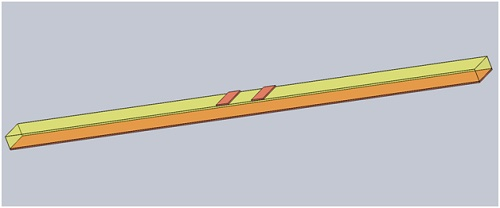Figure 6 - 1/20 of the structure is modeled for AC Magnetic analysis

Similar to the above Magnetostatic analysis, the two traces are modeled as coils.  In order to account for the skin depth in the AC resistance calculation, the coils are modeled as solids, i.e. wound coils do not support eddy current.  Figure 7 shows the AC resistance for frequencies from 1 KHz to 1 MHz computed by EMS and compared to Reference .   Whereas Figure 8 shows the AC inductance results and comparison for the same frequency range.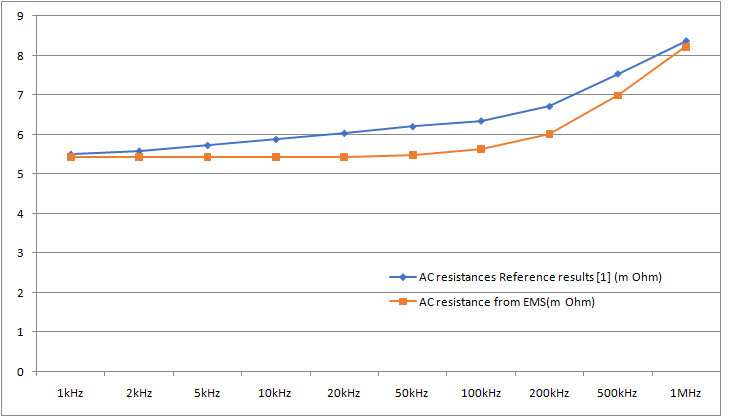Figure 7 - AC resistance computed by EMS and compared to Reference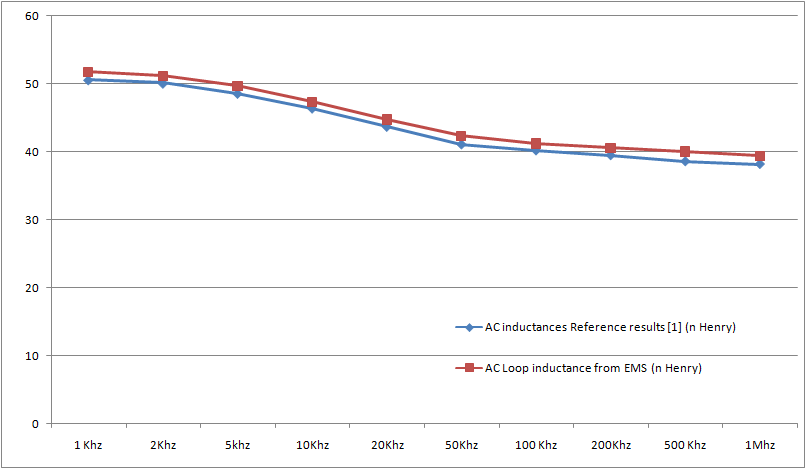Figure 8 - AC inductance computed by EMS and compared to Reference 

## Conclusion

Clearly, EMS compares well with the capacitance, AC and DC inductance and resistance published results  for PCB structures.  Hence EMS can readily be used to easily and quickly extract parasitic parameters for PCB and electronic structures which, in turn, can be used to build high frequency circuit models.

## References

 Jingen Qian, “RF Models for Active IPEMs”, MS Thesis in Electrical Engineering,  Virginia Polytechnic Institute and State University, January 31, 2003.